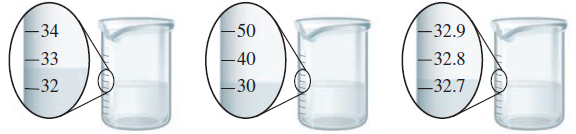# Problem: The beakers shown below have different precisions.a. Label the amount of water in each of the three beakers to the correct number of significant figures.b. Is it possible for each of the three beakers to contain the exact same amount of water? If no, why not? If yes, did you report the volumes as the same in part a? Explain.

###### FREE Expert Solution

For this problem, we're being asked if is it possible for each of the three beakers to contain the exact same amount of water

84% (263 ratings)###### Problem Details

The beakers shown below have different precisions.a. Label the amount of water in each of the three beakers to the correct number of significant figures.

b. Is it possible for each of the three beakers to contain the exact same amount of water? If no, why not? If yes, did you report the volumes as the same in part a? Explain.

Frequently Asked Questions

What scientific concept do you need to know in order to solve this problem?

Our tutors have indicated that to solve this problem you will need to apply the Significant Figures: Precision in Measurements concept. You can view video lessons to learn Significant Figures: Precision in Measurements. Or if you need more Significant Figures: Precision in Measurements practice, you can also practice Significant Figures: Precision in Measurements practice problems.

What professor is this problem relevant for?

Based on our data, we think this problem is relevant for Professor Achari's class at BU.

What textbook is this problem found in?

Our data indicates that this problem or a close variation was asked in Chemistry: An Atoms First Approach - Zumdahl Atoms 1st 2nd Edition. You can also practice Chemistry: An Atoms First Approach - Zumdahl Atoms 1st 2nd Edition practice problems.